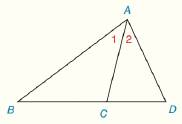Chapter 3.CR, Problem 14CR### Elementary Geometry for College St...

6th Edition
Daniel C. Alexander + 1 other
ISBN: 9781285195698

#### Solutions

Chapter
Section### Elementary Geometry for College St...

6th Edition
Daniel C. Alexander + 1 other
ISBN: 9781285195698
Textbook Problem
1 views

# Given: A C → bisects ∠ B A D Prove: A D > C DTo determine

To prove:

The side AD¯ is greater than the side CD¯.

Explanation

Given:

The given figure is,

Figure (1)

From figure (1).

Approach:

An angle bisector divides the angle in two congruent angles.

Opposite side of larger angle has the larger length in a triangle.

Approach:

Consider the triangle ΔABD,

From the given data,

The angle bisector AC¯ bisects BAD in two congruent angles m1 and m2. So,

m1=m2

Consider the triangle ΔABC,

Since, the measure of the exterior angle mACD of the triangle is the sum of measures of the two nonadjacent angles m1 and mB. Then,

mACD=m1+mB

Then, the exterior angle mACD is greater than the angle m1. So,

mACD>m1

Since, the angle m1 is equal to the angle m2 then,

mACD>m2

Since, the side opposite to larger angle in a triangle has the larger length

### Still sussing out bartleby?

Check out a sample textbook solution.

See a sample solution

#### The Solution to Your Study Problems

Bartleby provides explanations to thousands of textbook problems written by our experts, many with advanced degrees!

Get Started

#### Find more solutions based on key concepts